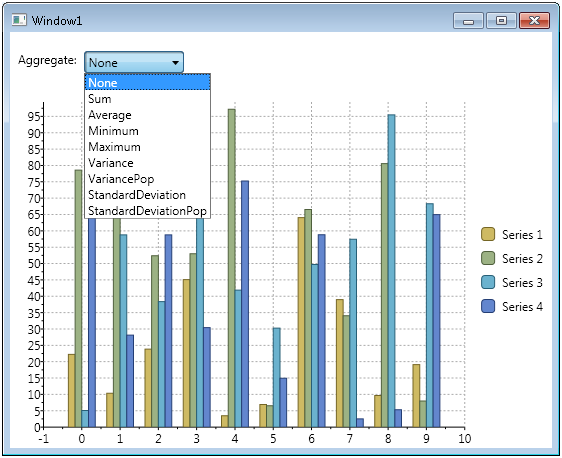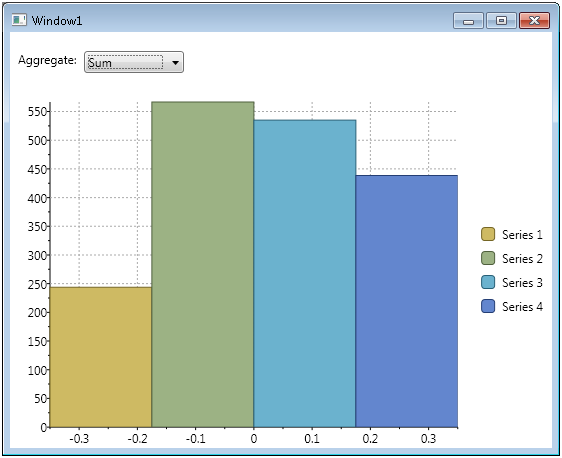﻿ What’s New: Chart Aggregates# What’s New: Chart Aggregates

The ComponentOne Chart for WPF and Silverlight has a new Aggregate feature which performs instant aggregation on the plotted data. By simply setting one property we can change the chart's view to calculate and display an aggregation, such as total count, average, sum and standard deviation. Let's see this in action. The following code will be for the WPF C1Chart. Create a new WPF application and replace the following XAML:

``````
<Grid>
<Grid.RowDefinitions>
<RowDefinition Height="60" />
<RowDefinition />
</Grid.RowDefinitions>
<StackPanel Margin="4" Orientation="Horizontal">
<TextBlock Margin="4" Text="Aggregate:"  />
<ComboBox Margin="4" x:Name="combo" >
<ComboBoxItem Content="None" />
<ComboBoxItem Content="Sum" />
<ComboBoxItem Content="Count" />
<ComboBoxItem Content="Average" />
<ComboBoxItem Content="Minimum" />
<ComboBoxItem Content="Maximum" />
<ComboBoxItem Content="Variance" />
<ComboBoxItem Content="VariancePop" />
<ComboBoxItem Content="StandardDeviation" />
<ComboBoxItem Content="StandardDeviationPop" />
</ComboBox>
</StackPanel>
<c1chart:C1Chart x:Name="chart" Grid.Row="1"></c1chart:C1Chart>
</Grid>

``````

Add a reference to ComponentOne Chart for WPF (C1.WPF.C1Chart), and add the following namespace in your XAML:

``````xmlns:c1chart="clr-namespace:C1.WPF.C1Chart;assembly=C1.WPF.C1Chart"
``````

Modify the following code:

``````
using C1.WPF.C1Chart;
public Window1()
{
InitializeComponent();

//Set Chart Aggregate to ComboBox value
combo.SelectionChanged += (s, e) =>
chart.Aggregate = (Aggregate)combo.SelectedIndex;

for(int i = 0; i < 4; i++)
{
var ds = new DataSeries();
ds.Label = "Series " + (i + 1).ToString();
ds.ValuesSource = CreateRandomValues(10);
}
}

Random rnd = new Random();
double[] CreateRandomValues(int cnt)
{
double[] vals = new double[cnt];
for (int i = 0; i < cnt; i++)
vals[i] = rnd.NextDouble() * 100;
return vals;
}

``````

Run the sample and notice we created four data series of random data.Select an aggregate option from the list and notice the C1Chart automatically calculates and displays the aggregated values.To reset the view simply select "None" from the aggregate list. The key line of code is setting the chart.Aggregate property.

``````chart.Aggregate = (Aggregate)combo.SelectedIndex;
``````

The Aggregate property takes an enum type of C1Chart.Aggregate, which contains 10 possible values: None, Sum, Count, Average, Minimum, Maximum, Variance, VariancePop, StandardDeviation and StandardDeviationPop. This property also exists on the DataSeries object, so you could show different aggregations for each series. Check out a live demo of this feature in the 2010 Control Explorer.Greg Lutz